cancel
Showing results for
Did you mean:Anonymous
Not applicable

## hiding columns in Matrix

Hi there,

Is it possible to hide columns and keep the total in a matrix. You can do something similar in SSRS by just adding a total at the end of the report.

In the image below, can I hide "Opportunity" in the matrix and keep the total?

Thank you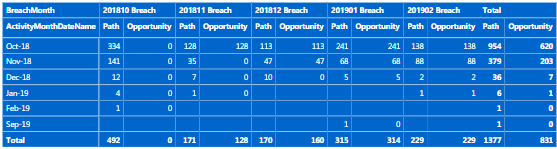1 ACCEPTED SOLUTIONAnonymous
Not applicable

Hi Frank,

I was able to solve the problem by turning off word wrap in the matrix setting and dragging the field I wanted to hide.16 REPLIES 16Frequent Visitor

Hi,

If you don't want to see particular column for different categories in the matrix then there is one solution.
For example,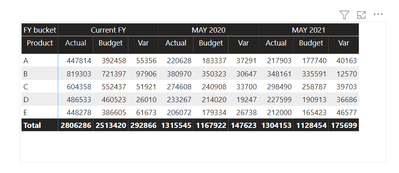From the above matrix and I want to see only
Current FY -> Actual, Budget
May 2020 -> Actual
May 20201 -> Actual, Budget and Var

So, for this I have created one another table where I am taking only those columns which I want to see in the matrix. As shown below

Categroy Table =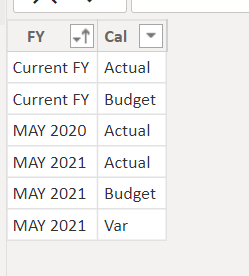You can make this table by just entering data and loading into the power bi.

Next step is to create relationship between Sales table and Category table.
My sales table has a calculated column named "FY Bucket".FY bucket =
SWITCH(TRUE(),

Sales[Month No] <= 5 && Sales[Year] = 2020,"MAY 2020",
Sales[Month No] <= 5 && Sales[Year] = 2021,"MAY 2021",
Sales[Month No] > 5 && Sales[Year] = 2021 || Sales[Year] = 2020 ,"Current FY","Others")

And now we will create the relationship between Category['FY'] & Sales['FY bucket']Note that, as both table has multiple values in the column it will display Many to Many relationship only.
To avoid many-to-many relationship you can create bridge table.

Next step is calculating Actual, Budget and Var for corresponding columns. Here, I am creating measure for that, it's formula is given below.

Sales Measure =
SWITCH(TRUE(),

SELECTEDVALUE('Category'[FY]) = "Current FY" && SELECTEDVALUE('Category'[Cal]) = "Actual",
CALCULATE(SUM(Sales[Actual]),
Sales[FY bucket] = "Current FY"),

SELECTEDVALUE('Category'[FY]) = "Current FY" && SELECTEDVALUE('Category'[Cal]) = "Budget",
CALCULATE(SUM(Sales[Budget]),
Sales[FY bucket] = "Current FY"),

SELECTEDVALUE('Category'[FY]) = "MAY 2020" && SELECTEDVALUE('Category'[Cal]) = "Actual",
CALCULATE(SUM(Sales[Actual]),
Sales[FY bucket] = "MAY 2020"),

SELECTEDVALUE('Category'[FY]) = "MAY 2021" && SELECTEDVALUE('Category'[Cal]) = "Actual",
CALCULATE(SUM(Sales[Actual]),
Sales[FY bucket] = "MAY 2021"),

SELECTEDVALUE('Category'[FY]) = "MAY 2021" && SELECTEDVALUE('Category'[Cal]) = "Budget",
CALCULATE(SUM(Sales[Budget]),
Sales[FY bucket] = "MAY 2021"),

SELECTEDVALUE('Category'[FY]) = "MAY 2021" && SELECTEDVALUE('Category'[Cal]) = "Var",
CALCULATE(SUM(Sales[Var]),
Sales[FY bucket] = "MAY 2021")
)

Put your measure as Values into the matrix.
And here is the result:Thank You! I hope you will like it.Frequent Visitor

Hi everyone,

This is a great solution!

For my situation though, I have a slightly different scenario.

I wish to show as null/blank for the "target" column values only when the third row in the matrix table is drilled down/shown.

This is because the target only applies to the first two rows, and is not applicable to the third row.Would anyone know if it is possible?

Thank you!Community Support

Hi @Anonymous,

There is no such an visibility option that supports to conditionally hide/show column in Matrix. One option is to hide or show the values in the column by creating measures, but column headers are still appeared even there is no data. Reference: https://community.powerbi.com/t5/Desktop/How-can-i-hide-Column/td-p/110087

Regards,

Frank

Community Support Team _ Frank
If this post helps, then please consider Accept it as the solution to help the others find it more quickly.Anonymous
Not applicable

Thank you Frank, isnt completely what am looking for but thats very close to it. Thanks once againCommunity Support

Hi @Anonymous,

Does that make sense? If so, kindly mark my answer as a solution to close the case.

Regards,
Frank

Community Support Team _ Frank
If this post helps, then please consider Accept it as the solution to help the others find it more quickly.Anonymous
Not applicable

Hi Frank,

I was able to solve the problem by turning off word wrap in the matrix setting and dragging the field I wanted to hide.New Member

If we squeeze columns, that will hide that column from report users but users who are exporting it to excel will still sble to view the hidden fields. Is there a way to hide from exported file as well.Frequent Visitor

hi

iam facing the same issue but iam not able to find the word wrap filed in the latest version of power bi desktop can please suggest me which field i need to see.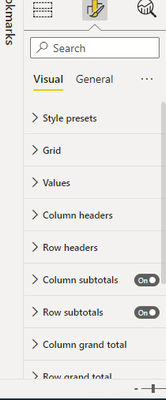Frequent Visitor

It is called Text Wrap now--the easiest way I'm finding to deal with the new layout is using the search bar in the formatting section.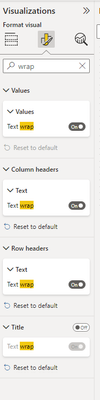Helper III

Hi,

I have similar situation-want to hard hightlighted in yellow color column. Want to see total. I try to follow your solution but lost.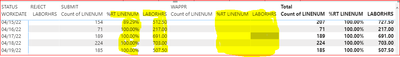under which categary I should go for "turning off word wrap " and dragging fieldThanksFrequent Visitor

hi

iam also facing the same issue can you please help me .i need to hide the order cases i want ordercases in total field.below i have marked i need to hide .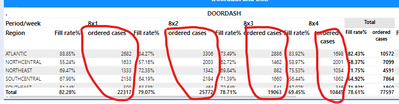Frequent Visitor

Someone else will probably have a better solution but this reminds me of one that I had a problem with because I needed 3 year's sales but only current year's budget.   I found the cleanest solution was to write measures for each year and one for the budget then put them into a table.   Had the advantage of giving me measures I could use for other calculations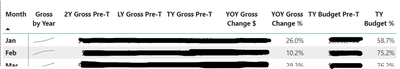So I think you could write a CALCULATE for each of your fill rates and then a total for your cases to build something similar. 🙂Frequent Visitor

Hi,

how to write the calculate function fillrate is the calculated measure and ordercases is a column periods also columns .

fillrate=sum of ordered cases/shipped cases

i have calculatedFrequent Visitor

I'm not great at DAX but this is what I would try.  Essentially you can use CALCULATE as a filter. You would need a new measure for each of the fill rate columns you want and you can build it using the fillrate you already did.  I'm not sure what the name of the column field is so...

8x1 Fill Rate=CALCULATE(fillrate, [Field]="8x1")

8x2 Fill Rate=CALCULATE(fillrate, [Field]="8x2")

8x3 Fill Rate=CALCULATE(fillrate, [Field]="8x3")

8x4 Fill Rate=CALCULATE(fillrate, [Field]="8x4")

Then you would need to make a measure for your Ordered Cases (not sure if you have any filters on cases but if so you could use calculate to get it right).

Ordered Cases Total = SUM[ordered cases]

If you want the Total Fill Rate at the end you will need one more measure

Total Fill Rate=CALCULATE(fillrate)

Then drop all the measures onto a table in the order you want to see them.   Depending on how your calculations work with your data it can take some fine tuning.  Hopefully, that works--like I said I'm no expert lol.Frequent Visitor

Just a note to say thank you.  This solved a issue that was driving me insaneImpactful Individual

Nice little workaround with the hiding of the column. 👍  Helped me out.